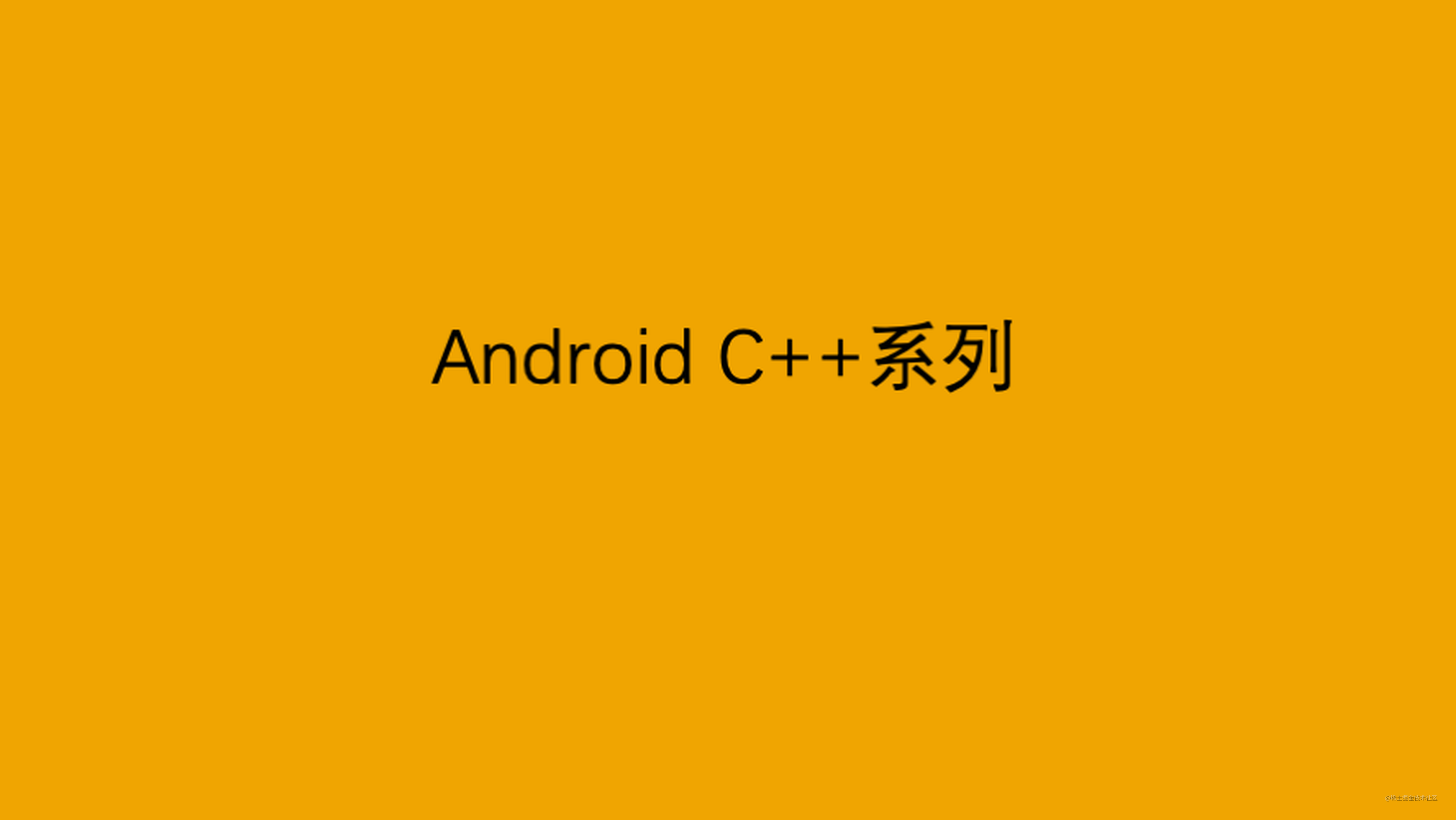# Android C++系列：函数返回值注意事项## 1. 背景

``````bool findChar(const string &str, const char c){
auto size = str.size();
for(decltype(size) i = 0; i < size; i++){
if(str[i] == c){
return true;
}
}
}

## 2. 理解值是如何被返回的

const string *getStr(){

​ string ret;

​ return ret; //返回局部对象的引用

​ //return "Hello"; //Hello是局部临时变量

}

## 3. 返回类类型的函数

``````std::vector<std:string> strs;
strs.begin().size();

## 4. 返回左值

``````int &getVal(int &i){
return i;
}
void main(){
int value = 100;
getVal(value) = 200;
}

## 5. 返回列表

C++11中允许我们使用花括号包围的值的列表当返回值，初始化临时的vector：

``````std::vector<int> getValues(){
return {1, 2,3,4};
}

## 6. 返回数组指针

``````typedef int arrT;//等价于using arrT = int;
arrT* fun(int i);

arrT是含有10个整数的数组的别名。

Android

Android